# Postprocessing¶

The postprocessing stage of a script processes the data from a finished simulation, for example plotting or exporting the value of the subjects’ state variables (v-values or w-values).

The postprocessing commands are the following:

Command

Description

Affected by parameters

@figure

Creates a figure window

None

Creates a subplot within the current figure window

None

@legend

Creates a legend for all plotted lines in the current subplot

None

@plot

Generic plot command

@vplot

Plots a v-value

@vssplot

Plots a vss-value (only in the
Rescorla-Wagner mechanism

@pplot

Plots the probabilitiy to respond with a
certain behavior to a certain stimulus

@wplot

Plots a w-value

@nplot

Plots the number of occurrences of a certain
stimulus/behavior or a chain
stimulus->behavior->stimulus->... or
behavior->stimulus->behavior->...

@export

Generic export commmand

filename and same as @plot

@vexport

Exports the values of a @vplot

filename and same as @vplot

@vssexport

Exports the values of a @vssplot

filename and same as @vssplot

@pexport

Exports the values of a @pplot

filename and same as @pplot

@wexport

Exports the values of a @wplot

filename and same as @wplot

@nexport

Exports the values of a @nplot

filename and same as @nplot

@hexport

Exports the history of stimuli and
responses

The below plots show the components of a plot window:## @figure¶

Creates a new figure window.

Syntax:

@figure
@figure figure_title
@figure figure_title figure_parameters
@figure figure_parameters


where

• figure_title is an optional title of the figure (any string),

• figure_parameters is an optional specification of Matplotlib Figure parameters controlling figure size, colors, etc. of the format {param1:value1, param2:value2, ...}. See figure_parameters for the supported figure parameters.

It is also possible to specify the subplot grid in parenthesis after @figure:

@figure(1,3) [figure_title] [figure_parameters]
@subplot
@plot ...  # This plot ends up in subplot 131
@subplot
@plot ...  # This plot ends up in subplot 132
@subplot
@plot ...  # This plot ends up in subplot 133


as an alternative form of:

@figure [figure_title] [figure_parameters]
@subplot 131
@plot ...  # This plot ends up in subplot 131
@subplot 132
@plot ...  # This plot ends up in subplot 132
@subplot 133
@plot ...  # This plot ends up in subplot 133


Note

If a plot command or a @subplot command is issued without a preceding @figure command, a default figure window will automatically be created.

### Example 1¶

@figure


creates a default figure without title and with the default Matplotlib Figure parameters.

### Example 2¶

@figure This is the title


creates a figure with the title “This is the title” and with the default Matplotlib Figure parameters: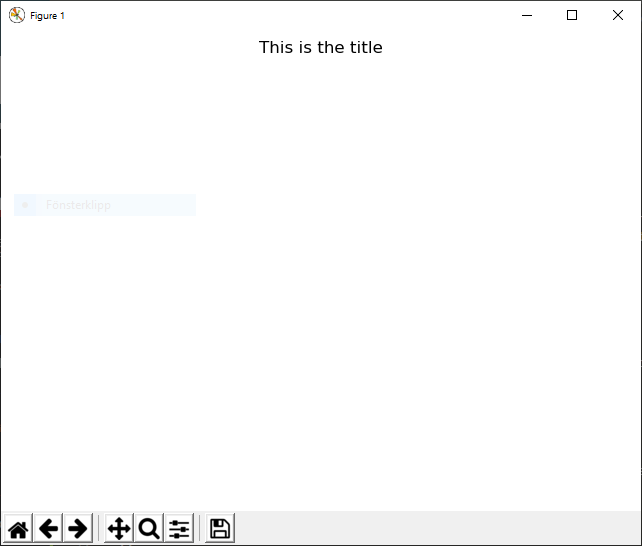### Example 3¶

@figure This is the title {'edgecolor':'blue','linewidth':10,'facecolor':'red'}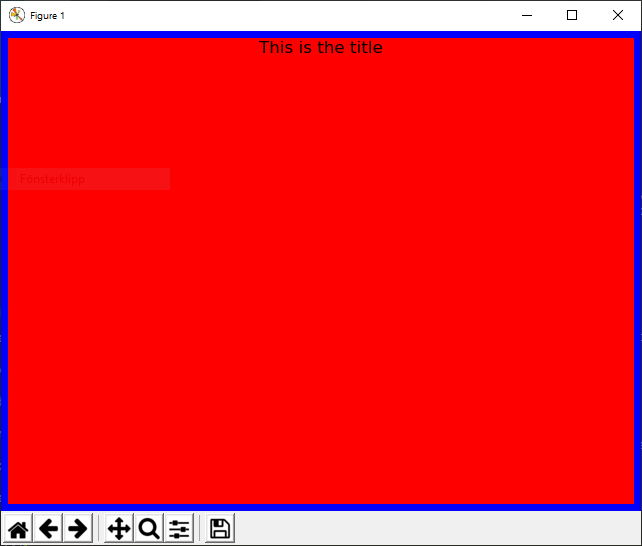creates a figure with the title “This is the title” with a blue edge (width 10) and red background:

### Example 4¶

@figure {'edgecolor':'green','linewidth':20,'facecolor':'magenta'}


creates a figure without title and with a green edge (width 5) and magenta background: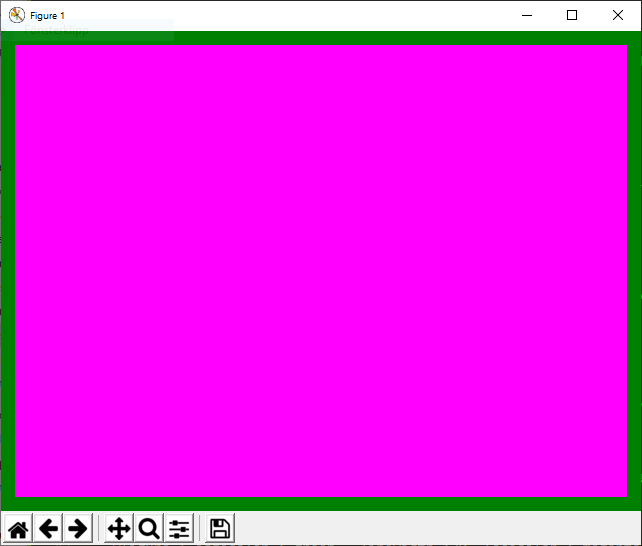## @subplot¶

The command @subplot creates an axis within a figure in which to make plots using any of the plotting commands (@vplot, @wplot, etc.)

Syntax:

@subplot pos
@subplot pos subplot_title
@subplot pos subplot_title subplot_parameters

• pos describes the position of the subplot within the figure. It is either a 3-digit integer (nrows)(ncols)(index) (for example 223), or three comma-separated integers in parenthesis (nrows, ncols, index) (for example (2,2,3)). The subplot will take the position index on a grid with nrows rows and ncols columns. index starts at 1 in the upper left corner and increases to the right.

• subplot_parameters is an optional specification of Matplotlib Subplot parameters controlling for example the background color, in the format {param1:value1, param2:value2, ...}. See subplot parameters for the supported subplot parameters.

Useful Subplot parameters to control the appearance of the subplot include

• xlim (The min- and max value of the x-axis)

• ylim (The min- and max value of the y-axis)

• xlabel (The label of the x-axis)

• ylabel (The label of the y-axis)

stated as:

{'xlim':[xmin,xmax], 'ylim':[ymin,ymax], 'xlabel':'x-axis label', 'ylabel':'y-axis label'}


where xmin, xmax, ymin, and ymax are numbers.

Note

If a plot command is issued without a preceding @subplot command, a default subplot axis will automatically be created (corresponding to @subplot 111).

### Example¶

@figure {'edgecolor':'black','linewidth':20,'facecolor':'grey'}
@subplot 221 Subplot 221 {'facecolor':'red'}
@subplot 222 Subplot 222 {'facecolor':'green'}
@subplot 223 Subplot 223 {'facecolor':'blue'}
@subplot 224 Subplot 224 {'facecolor':'yellow'}## @panel¶

Same as @subplot.

## @legend¶

The command @legend creates a legend with labels for each plotted curve in the last created subplot. For the labels, the legend uses default descriptive labels (that depends on the type of plot), but these may be overridden by custom labels using the label parameter to the plotting commands.

Syntax:

@legend
@legend legend_parameters


where legend_parameters is an optional specification of Matplotlib Legend parameters controlling for example the frame and background colors, in the format {param1:value1, param2:value2, ...}. See legend parameters for the supported legend parameters.

### Example 1¶

Running this script:

n_subjects        = 100
mechanism         = sr
behaviors         = response, no_response
stimulus_elements = background, s, reward
start_v           = default:1
alpha_v           = 0.1
u                 = reward:3, default:0

@PHASE training stop: s==100
START_TRIAL s          | response: REWARD | NO_REWARD
REWARD      reward     | START_TRIAL
NO_REWARD   background | START_TRIAL

@run training

xscale = s

# Postprocessing commands
@subplot 111 {'xlabel':'Number of exposures to s', 'ylabel':'v-value'}
@vplot s->response
@vplot s->no_response {'label':'custom label'}
@legend


creates the following plot: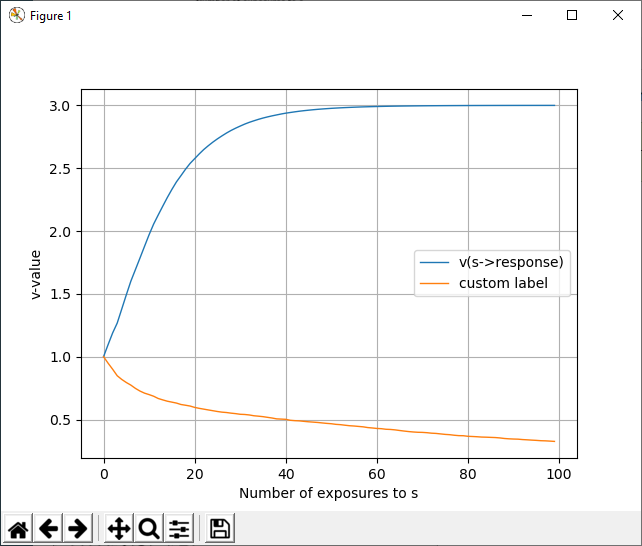### Example 2¶

With the same simulation as in Example 1 but the following postprocessing commands:

@vplot s->response {'linestyle':'--', 'color':'black'}
@vplot s->no_response {'label':'my custom label', 'marker':'o', 'color':'red','markevery':10}
@legend {'title':'my legend title', 'fontsize':20, 'facecolor':'grey'}


creates the following figure:## @plot¶

The command @plot plots one or several expressions that include v-values (as plotted with @vplot), w-values (as plotted with @wplot), p-values (as plotted with @pplot), vss-values (as plotted with @vssplot), and n-values (as plotted with @nplot).

The plotted entity can be an arithemtic expression of these values. For example:

@plot 2*v(s->b) - w(s)
@plot n(s1->b1->s2->b2) / n(s1->b1->s2)


To plot several expressions in the same @plot command, separate them by semicolon:

@plot v(s->b); p(s->b) * p(s1->b1); sin(n(s))


which is the same as:

@plot v(s->b)
@plot p(s->b) * p(s1->b1)
@plot sin(n(s))


Note that

• @plot v(s->b) is the same as @vplot s->b

• @plot p(s1,s2->b) is the same as @pplot s1,s2->b

• @plot w(s) is the same as @wplot s

The arithemtic expression may include the below operators and functions:

• +, -, *, /, **

• the trigonometric functions sin, cos, tan

• the inverse trigonometric functions asin, acos, atan

• the hyperbolic functions sinh, cosh, tanh

• the inverse hyperbolic functions asinh, acosh, atanh

• round, ceil, floor

• exp, log, log10, sqrt

Note that wildcards (*) cannot be used in @plot.

## @vplot¶

The command @vplot plots one or several v-values (the associative strength between a stimulus element and a behavior).

Syntax:

@vplot e->b
@vplot e->b line_parameters


plots the associative strength between the stimulus element e and the behavior b, and line_parameters are Line2D parameters. To plot several lines in the same subplot, use:

@vplot e1->b1; e2->b2; ...
@vplot e1->b1; e2->b2; line_parameters


Wildcards are also allowed. If:

stimulus_elements = s1, s2
behaviors = b1, b2, b3


then:

@vplot s1->*


is short for:

@vplot s1->b1
@vplot s1->b2
@vplot s1->b3


and:

@vplot *->*


is short for:

@vplot s1->b1
@vplot s1->b2
@vplot s1->b3
@vplot s2->b1
@vplot s2->b2
@vplot s3->b3


## @vssplot¶

The command @vssplot plots one or several vss-value (the associative strength between two stimulus elements).

Note

Only the Rescorla-Wagner learning mechanism has vss-values, so the command @vssplot is only applicable when mechnism=rw.

Syntax:

@vssplot e1->e2
@vssplot e1->e2 line_parameters


plots the associative strength between the stimulus elements e1 and e2, and line_parameters are Line2D parameters. To plot several lines in the same subplot, use:

@vssplot e1->e2; e3->e4; ...
@vssplot e1->e2; e3->e4; ... line_parameters


Wildcards are also allowed. If:

stimulus_elements = s1, s2, s3


then:

@vssplot s1->*


is short for:

@vssplot s1->s1
@vssplot s1->s2
@vssplot s1->s3


and:

@vssplot *->*


is short for:

@vssplot s1->s1
@vssplot s1->s2
@vssplot s1->s3
@vssplot s2->s1
@vssplot s2->s2
@vssplot s2->s3
@vssplot s3->s1
@vssplot s3->s2
@vssplot s3->s3


## @pplot¶

The command @pplot plots the probability of responding with a certain behavior to a certain stimulus, according to The decision function.

Syntax:

@pplot s->b
@pplot s->b line_parameters


plots the probability of responding with b to the stimulus s, and line_parameters are Line2D parameters. To plot several lines in the same subplot, use:

@pplot s1->b1; s2->b2; ...
@pplot s1->b1; s2->b2; ... line_parameters


Note that stimuli consisting of several stimulus elements (compound stimuli) are supported, and that an intensity may be specified to each element, for example:

@pplot s1[0.5],s2[0.6]->b1; s2->b2; ...
@pplot s1[0.5],s2[0.6]->b1; s2->b2; ... line_parameters


plots (1) the probabililty of responding with b1 to the compound stimulus s1[0.5],s2[0.6], and (2) the probabililty of responding with b2 to the stimulus s2 in the same subplot.

Wildcards are also allowed. If:

stimulus_elements = s1, s2
behaviors = b1, b2, b3


then:

@pplot s1->*


is short for:

@pplot s1->b1
@pplot s1->b2
@pplot s1->b3


and:

@pplot *->*


is short for:

@pplot s1->b1
@pplot s1->b2
@pplot s1->b3
@pplot s2->b1
@pplot s2->b2
@pplot s3->b3


## @wplot¶

The command @wplot plots one or several w-values (the conditioned reinforcement for a stimulus element) for the mechanisms that support this (A-learning and Actor-critic).

Syntax:

@wplot e
@wplot e line_parameters


plots the conditioned reinforcement value for the stimulus element e, and line_parameters are Line2D parameters. To plot several lines in the same subplot, use:

@wplot e1; e2; ...
@wplot e1; e2; e3; ... line_parameters


Wildcards are also allowed. If:

stimulus_elements = s1, s2, s3


then:

@wplot *


is short for:

@wplot s1
@wplot s2
@wplot s3


## @nplot¶

The command @nplot plots the number of occurrences of a stimulus, a behavior, or a chain stimulus->behavior->stimulus->... or behavior->stimulus->behavior->... in the history of stimulus-response pairs during the simulation, and line_parameters are Line2D parameters.

Syntax:

@nplot stimulus
@nplot behavior
@nplot stimulus1->behavior1->stimulus2->...
@nplot behavior1->stimulus1->behavior2->...
@nplot ... line_parameters


where stimulus is a stimulus, i.e. in general a comma-separated list of stimulus elements, behavior is a behavior, and line_parameters are Line2D parameters.

To plot several lines in the same subplot, use:

@nplot x->y->z->...; a->b->c->...


### cumulative¶

The parameter cumulative can have the values on or off and affects @nplot input in the following way:

If cumulative is off:

• If xscale is all: At each point (stimulus or response) in the history, the y-value is 1 if input matches the simulation sequence at that point, and 0 otherwise.

• If xscale is not all: The y-value shows the number of occurrences of input between each point (stimulus or response) in the history where xscale matches the simulation sequence. For example, the y-value at $$x=1$$ is the number of occurrences of input between the first and the second occurrence of input, and the y-value at $$x=2$$ is the number of occurrences of input between the second and the third, etc.

If cumulative is on, the values above are added cumulatively at each x-value. For example, the value at $$x=4$$ shows the sum of the values at $$x=0$$, $$x=1$$, $$x=2$$, and $$x=3$$.

Note

With multiple subjects and subject=average the plotting commands (including @nplot) renders the average y-value of all subjects, which means that the y-value can be between 0 and 1 even if the y-value for each individual subject is 0 or 1.

### Example¶

With the combination xscale=s (for some stimulus s), cumulative=off, and n_subjects>1:

@pplot s->b


shows, at each exposure of s, the probability to respond with the behavior b to the stimulus s, whereas:

@nplot s->b


shows, at each exposure of s, the empirical average (over all subjects) fraction of times when an subject responded with b to s in the simulation:

n_subjects        = 500
mechanism         = SR
behaviors         = respond,ignore
stimulus_elements = s, reward
start_v           = s->ignore:0, default:-1
alpha_v           = 0.1
beta              = 1
u                 = reward:2, default:0

@phase instrumental_conditioning stop:s=100
STIMULUS    s          | respond: REWARD  | STIMULUS
REWARD      reward     | STIMULUS

@run instrumental_conditioning

xscale     = s
cumulative = off

@subplot 111 {'xlabel':'Number of exposures to s', 'ylabel':'Proportion/probability of response to s'}
@nplot s->respond
@pplot s->respond
@legend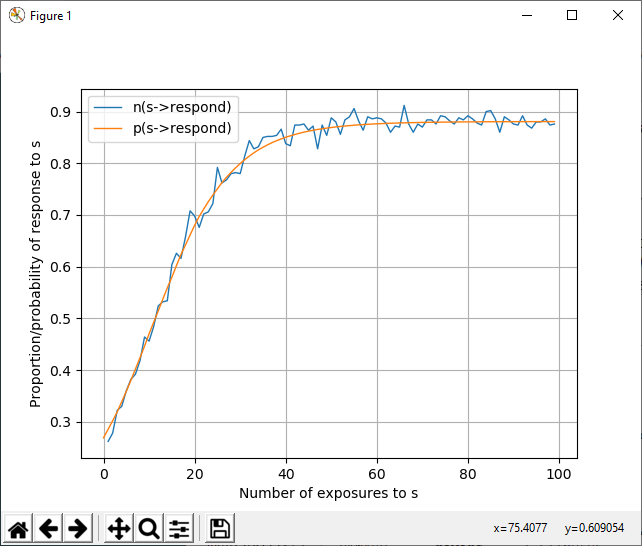## Line2D parameters¶

The input line_parameters to the plotting commands is an optional specification of Matplotlib Line2D parameters controlling for example line color and line style, in the format {param1:value1, param2:value2, ...}. See Line2D parameters for the supported Line2D parameters.

Use the Line2D parameter label to set a custom label for the legend (produced with @legend) to the plotted line.

Useful Line2D parameters to control the appearance of the plot include color, linestyle, marker, markevery.

## Exporting data to file¶

The data-exporting commands @export, @vexport, @wexport, @pexport, and @nexport exports to a csv-file the data that is plotted with the plotting commads @plot, @vplot, @wplot, @pplot, and @nplot, respectively. Therefore the same parameters that affect the plot also affect the exported data (for example xscale, phases, subject, runlabel, etc.)

In addition, the parameter filename is required to all exporting commands.

The command @hexport simply exports the sequence of alternating stimuli and responses for the @run specified with the parameter runlabel. There is one sequence for each subject (regardless of the value of the parameter subject). The exported data with @hexport is not affected by any other parameter.

The destination file is specified with the parameter filename.

Note

If the specified file already exists, the existing file will be overwritten without warning.

### Format of the csv-file¶

The data export commands @vexport, @wexport, @pexport, and @nexport exports the data as a csv-file with two or more columns. The first column, with title “x” contains step numbers (corresponding to the x-axis in the corresponding plot command). The second column onwards contains the data for the specified quantity for each subject (controlled by the subject parameter). For example, after the commands:

n_subjects = 5
subject = all
filename = my_file.csv
@vexport s->b


the first line, containing the headings, in the file my_file.csv looks like this:

"x","v(s->b) subject 0","v(s->b) subject 1","v(s->b) subject 2","v(s->b) subject 3","v(s->b) subject 4"


If instead subject = average, the first line will look like this:

"x","v(s->b)"


The remaining lines contain comma-separated values for the quantities in the heading. An example csv-file exported with @vexport s->response may look like this:

"x","v(s->response) subject 0","v(s->response) subject 1","v(s->response) subject 2","v(s->response) subject 3","v(s->response) subject 4"
0,1,1,1,1,1
1,1.2,1.2,1.2,1,1
2,1.2,1.2,1.2,1,1
3,1.38,1.2,1.38,1.2,1.2
4,1.38,1.2,1.38,1.2,1.2
5,1.38,1.38,1.5419999999999998,1.38,1.2
6,1.38,1.38,1.5419999999999998,1.38,1.2
7,1.5419999999999998,1.38,1.6877999999999997,1.38,1.38
8,1.5419999999999998,1.38,1.6877999999999997,1.38,1.38
9,1.6877999999999997,1.5419999999999998,1.8190199999999999,1.5419999999999998,1.38


The @hexport command exports the sequence of alternating stimuli and responses for each subject, and has therefore a different format. See @hexport.

## @export¶

@export exports the data plotted by @plot to the csv-file specified with the parameter filename.

Syntax:

filename = my_file.csv
@export v(s1->b) + v(s2->b)


The file name can be specified at the end of the command instead of using the parameter filename:

Note that wildcards (*) cannot be used in @export.

## @vexport¶

@vexport exports the data plotted by @vplot to the csv-file specified with the parameter filename.

Syntax:

filename = my_file.csv
@vexport e->b
@vexport e1->b1; e2->b2; ...
@vexport e->*
@vexport *->b
@vexport *->*


The file name can be specified at the end of the command instead of using the parameter filename:

@vexport e->b my_file.csv
@vexport e1->b1; e2->b2; ... my_file.csv
@vexport e->* my_file.csv
@vexport *->b my_file.csv
@vexport *->* my_file.csv


## @vssexport¶

@vssexport exports the data plotted by @vssplot to the csv-file specified with the parameter filename.

Note

Only the Rescorla-Wagner learning mechanism has vss-values, so the command @vssplot is only applicable when mechanism=rw.

Syntax:

filename = my_file.csv
@vssexport e1->e2
@vssexport e1->e2; e3->e4; ...
@vssexport e1->*
@vssexport *->e1
@vssexport *->*


The file name can be specified at the end of the command instead of using the parameter filename:

@vssexport e1->e2 my_file.csv
@vssexport e1->e2; e3->e4; ... my_file.csv
@vssexport e1->* my_file.csv
@vssexport *->e1 my_file.csv
@vssexport *->* my_file.csv


## @pexport¶

@pexport exports the data plotted by @pplot to the csv-file specified with the parameter filename.

Syntax:

filename = my_file.csv
@pexport s->b
@pexport s1->b1; s2->b2; ...
@pexport s->*
@pexport *->b
@pexport *->*


The file name can be specified at the end of the command instead of using the parameter filename:

@pexport s->b my_file.csv
@pexport s1->b1; s2->b2; ... my_file.csv
@pexport s->* my_file.csv
@pexport *->b my_file.csv
@pexport *->* my_file.csv


## @wexport¶

@wexport exports the data plotted by @wplot to the csv-file specified with the parameter filename.

Syntax:

filename = my_file.csv
@wexport e
@wexport e1; e2; ...
@wexport *


The file name can be specified at the end of the command instead of using the parameter filename:

@wexport e my_file.csv
@wexport e1; e2; ... my_file.csv
@wexport * my_file.csv


## @nexport¶

@nexport exports the data plotted by @nplot to the csv-file specified with the parameter filename.

Syntax:

filename = my_file.csv
@nexport stimulus
@nexport behavior
@nexport stimulus1->behavior1->stimulus2->...
@nexport behavior1->stimulus1->behavior2->...
@nplot x->y->z->...; a->b->c->...


The file name can be specified at the end of the command instead of using the parameter filename:

@nexport stimulus my_file.csv
@nexport behavior my_file.csv
@nexport stimulus1->behavior1->stimulus2->... my_file.csv
@nexport behavior1->stimulus1->behavior2->... my_file.csv
@nplot x->y->z->...; a->b->c->... my_file.csv


## @hexport¶

The @hexport command exports a csv-file with three or more columns. Column 1 contains step numbers. Columns 2 and 3 contains the stimulus and response, respectively, for subject 1. Column 4 and 5 contains the stimulus and response, respectively, for subject 2, etc:

"step","stimulus subject 0","response subject 0","stimulus subject 1","response subject 1","stimulus subject 2","response subject 2",...


Syntax:

filename = my_file.csv
@hexport


or:

@hexport my_file.csv


Note

All subjects are always included in the output file from @hexport.